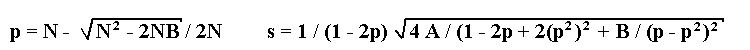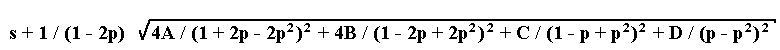## Equations for estimation of recombination fraction between a marker and a translocation breakpoint

 Kosterin, O.E., Pukhnacheva, N.V., Gorel, F.L. and Berdnikov, V.A. Institute of Cytology & Genetics Novosibirsk 630090 Russia

We present here equations for estimation of recombination fraction between a marker and a translocation breakpoint, followed by pollen fertility versus semisterility, in F2 progenies with the maximum likelihood method.
If a lethal is linked to a recessive allele a of a marker, then the maximum likelihood estimation p of the recombination fraction and its standard deviation s will be determined by the following formulae:
1. A marker is codominant. There exist six phenotypic classes with respect to the marker and pollen fertility. Let us introduce the following designations: N, the total number of progeny; A, the sum of three major classes: homozygotes for the marker with fertile pollen and heterozygote with semisterile pollen; B, the sum of three minor classes: heterozygote with fertile pollen and homozygotes with semisterile pollen.
Solution of the maximum likelihood equation leads to the following formulae for estimations of recombination fraction p and its standard deviation s:2. The marker is dominant. Let us designate the four phenotypic classes existing as follows: A, dominant fertile; B, recessive fertile; C, dominant semisterile; D, recessive semisterile. The estimation p can be found by numerical solution of the equation

- 2A / (1 + 2p - 2p2) + 2B / (1 - 2p + 2p2) + C / (1 - p + p2) - D / (p - p2) = 0

while the standard deviation is